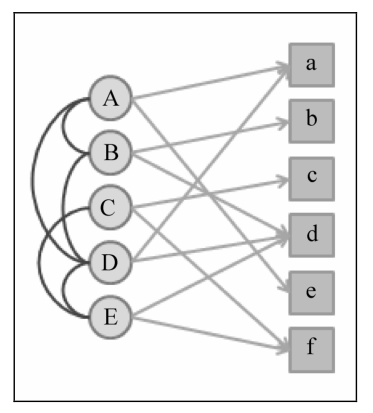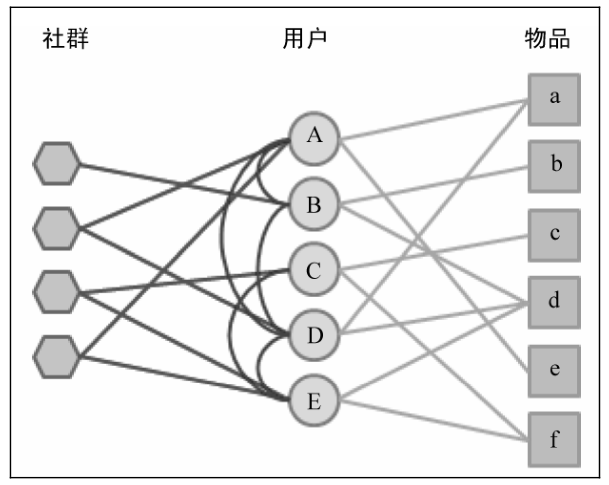# 《推荐系统实践》第6章:利用社交网络数据的推荐系统

1. 基于邻域的社会化推荐算法

$p_{u i}=\sum_{v \in \text { out }(u)} r_{v i}$

$p_{u i}=\sum_{v \in \text { out }(u)} w_{u v} r_{v i}$

$\text { familiarity }(u, v)=\frac{| \text { out }(u) \cap \operatorname{out}(v) |}{| \text { out }(u) \cup \operatorname{out}(v) |}$
$\text { similiarity }(u, v)=\frac{|N(u) \cap N(v)|}{|N(u) \cup N(v)|}$

1. 基于图的社会化推荐算法• 为每个用户维护一个消息队列，存储其推荐列表；
• 当一个用户喜欢一个物品后，就将记录写入关注该用户的推荐列表消息队列中；
• 当用户访问时，读出他的推荐列表消息队列，对于这个消息队列中的每个物品，重新计算该物品的权重。考虑物品在队列中出现的次数（行为数据），物品对应的用户和当前用户的熟悉程度（社交信息），物品的时间戳（上下文信息）。

3种基于社交网络的好友推荐算法：

• 对用户uv，通过共同好友计算相似度：

$w_{\text {out }}(u, v)=\frac{| \text { out }(u) \cap \operatorname{out}(v) |}{\sqrt{|\operatorname{out}(u)||\operatorname{out}(v)|}}$

• 指向用户的用户群的相似度：

$w_{\mathrm{in}}(u, v)=\frac{|\operatorname{in}(u) \bigcap \operatorname{in}(v)|}{\sqrt{\operatorname{in}(u)|| \operatorname{in}(v) |}}$

• 用户u中关注的用户有多少关注了v：

$w_{\text {out }, \text { in }}^{\prime}(u, v)=\frac{| \text { out }(u) \bigcap \operatorname{in}(v) |}{\sqrt{|\operatorname{out}(u)||\operatorname{in}(v)|}}$

©️2019 CSDN 皮肤主题: 精致技术 设计师: CSDN官方博客